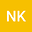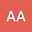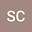Fuzzy Kinematic Finite-Fault Inversion: 1. Methodology
•••Navid KheirdastInternational Institute of Earthquake Engineering and Seismology (IIEES), International Institute of Earthquake Engineering and Seismology (IIEES)
Author ProfileAnooshiravan AnsariInternational Institute of Earthquake Engineering and Seismology (IIEES), International Institute of Earthquake Engineering and Seismology (IIEES)

Corresponding Author:a.ansari@iiees.ac.ir

Author Profile## Abstract

Finite-fault kinematic source inversions aim at resolving the spatio-temporal evolution of slip on a fault plane given ground motion recorded on the Earth’s surface. This type of inverse problem is inherently ill-posed and rank-deficient due to two main factors. First, the number of model parameters is typically greater than the number of observed data. The second issue is that small singular values result from the discretization of the physical rupture process, amplifying the effect of noise in the inversion. Consequently, we can find different slip distributions that fit the data equally well. This ill-posedness can be mitigated by decreasing the number of model parameters, hence improving their relationship to the observed data. In this article, we propose a fuzzy function approximation approach to describe the slip function. In particular, we use Adaptive Network-based Fuzzy Inference System (ANFIS) to find the most adequate discretization for the spatial variation of slip on the fault. The fuzzy basis functions and their respective amplitudes are optimized through hybrid-learning. We solve this earthquake source problem in the frequency domain, searching for independent optimal spatial slip distributions for each frequency. The approximated frequency-dependent spatial slip functions are then used to compute the forward relationship between slip on the fault and ground motion. The method is constrained through Tikhonov regularization, requiring a smooth spatial slip variation. We discuss how the number of model parameters can be decreased while keeping the inversion stable and achieving an adequate resolution. The proposed inversion methodology is tested using the SIV1-benchmark exercise.
Aug 2021Published in Journal of Geophysical Research: Solid Earth volume 126 issue 8. 10.1029/2020JB020770